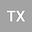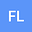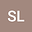Finite difference/finite element method for two-dimensional time and space fractional Bloch-Torrey equations with variable coefficients on irregular convex domains
•••• +1
• Tao Xu,
• Fa-Wang LIU,
• Shujuan Lv,
• V. Anh
Tao Xu
Beihang University Beijing

Corresponding Author:[email protected]

Author ProfileFa-Wang LIU
Queensland University of Technology
Author ProfileShujuan Lv
Beihang University
Author ProfileV. Anh
Swinburne University of Technology
Author Profile## Abstract

In magnetic resonance imaging of the human brain, the diffusion process of tissue water is considered in the complex tissue environment of cells, membranes and connective tissue. Models based on fractional order Bloch-Torrey equations can provide a new insights into further investigations of tissue structures and the microenvironment. In this paper, we consider new two-dimensional multi-term time and space fractional Bloch-Torrey equations with variable coefficients on irregular convex domains, which involves the Caputo time fractional derivative and the Riemann-Liouville space fractional derivative. An unstructured-mesh Galerkin finite element method is used to discretise the spatial fractional derivative, while for each time fractional derivative we use a novel $L1$ scheme on a temporal graded mesh. The stability and convergence of the fully discrete scheme are proved. Numerical examples are given to verify the efficiency of our method.